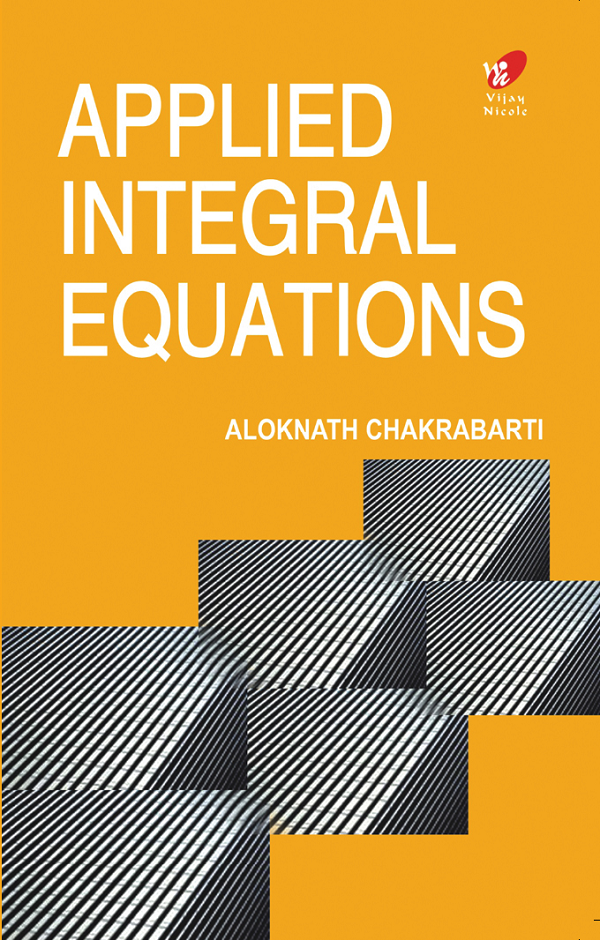Applied Integral Equations
Author(s):
Subject(s):
ISBN 9789394681842
Published Date
Pages 288
Description

Integral Equations arise naturally in engineering and Scientific applications modeled by initial value and boundary value problems. This book focuses mainly on the analytical treatment of linear integral equations of the first and second kind. The book will be of immense use to postgraduate students and research scholars of mathematics.

Cover
Title Page
Contents
List of Figures
Preface
1 OCCURRENCES AND EXAMPLES OF INTEGRAL EQUATIONS
1.1 Basic Definitions and Concepts
1.2 Occurrences of Integral Equations andExamples
1.2.1 Abel’s Problem
1.2.2 Initial Value Problems for Ordinary Differential Equations
1.2.3 Boundary Value Problems for Ordinary Differential Equations
1.2.4 Interesting Problems
1.2.5 Second Order Initial Value Problems and Boundary Value Problems of aSpecial Type
1.2.6 Two Special Boundary Value Problems Involving Partial Differential Equation
1.3 Exercises
2 SOME SPECIAL INTEGRAL EQUATIONS AND THEIR SOLUTIONS
2.1 Results on Integral Transforms
2.1.1 Laplace Transform
2.1.2 Fourier Transform
2.1.3 Mellin Transform
2.2 Use of Integral Transforms in Integral Equation
2.2.1 Volterra Equations of the First Kind
2.2.2 The Abel Integral Equation
2.2.3 The Stieltz Integral Equation
2.2.4 Fredholm Equations of the Second Kind .
2.2.5 The Fox Integral Equation
2.2.6 The Wiener–Hopf Integral Equation
2.3 Exercises
3 INTEGRAL EQUATIONS OF THE SECOND KIND
3.1 Degenerate Kernels
3.2 General Kernels
3.2.1 Continuous Kernels and Forcing Terms .
3.2.2 Square-Integrable Kernels and Forcing Terms
3.3 Exercises
4 INTEGRAL EQUATIONS WITH SYMMETRIC KERNELS
4.1 Some Basic Results
4.2 The Rayleigh–Ritz Method
4.3 Determination of Subsequent Eigenvalues of a Symmetric Kernel
4.4 The Hilbert–Schmidt Theory
4.5 Other Approximation Methods for Eigenvalues of Symmetric Kernels
4.5.1 The Trace Method
4.5.2 Kellogg’s Method
4.5.3 Galerkin’s Method
4.6 Exercises
5 SINGULAR INTEGRAL EQUATIONS WITH CAUCHY-TYPE KERNELS
5.1 Occurrence of Singular Integral Equations
5.2 Some Important Theorems and Results in Complex Function Theory
5.3 Solution of Singular Integral Equations Involving Closed Contours
5.4 Solution of Singular Integral Equations involving Open Arcs
5.5 Exercises
6 ON NUMERICAL METHODS FOR INTEGRAL EQUATIONS
6.2 Other Numerical Methods
6.2.1 Kernel Approximation Method
6.2.2 The Least Squares Method
6.3 Exercises
7 WORKED OUT PROBLEMS
7.1 Problems from Chapter 1
7.2 Problems from Chapter 2
7.3 Problems from Chapter III
7.4 Problems from Chapter IV
7.4.1 Special Section: Boundary Value Problems, Green’s Functions, Integral Equations with Positive Symmetric Kernels
7.5 Problems from Chapter V
7.6 Problems from Chapter VI
Bibliography

Dr Aloknath Chakrabarti is a retired professor of Mathematics, Indian Institute of Science, Bangalore, India. He is at present a Visiting Professor, Department of Mechanical Engineering, University of Hong Kong, Pokfulam Road, Hong Kong. He has more than four decades of rich experience in teaching and research on various theories of Mathematics. A Gold Medalist in M.Sc (Applied Mathematics) from Calcutta University, his areas of special interest are Integral Transforms, Integral Equations, Elasticity, Fluid Mechanics, Diffraction and Scattering Theory, The Weiner-Hopf Tehnique, and Numerical Analysis.Dr Chakrabarti has published numerous articles in reputed national and international journals, including two books titled Elements of Ordinary Differential Equations and Special Functions, published by Wiley Eastern Pvt. Ltd., (1991) and Water Wave Scattering by Barriers (along with Prof. BN Mandal), WIT Press, Southampton, Boston (2000). He is a Fellow of the National Academy of Sciences, India, a Fellow and Chartered Mathematician, Institute of Mathematics and its Applications, U.K., and a Fellow of the Wessex Institute of Great Britain.